# Test: Dot Product And Cross Product

## 30 Questions MCQ Test Engineering Mechanics | Test: Dot Product And Cross Product

Description
This mock test of Test: Dot Product And Cross Product for Mechanical Engineering helps you for every Mechanical Engineering entrance exam. This contains 30 Multiple Choice Questions for Mechanical Engineering Test: Dot Product And Cross Product (mcq) to study with solutions a complete question bank. The solved questions answers in this Test: Dot Product And Cross Product quiz give you a good mix of easy questions and tough questions. Mechanical Engineering students definitely take this Test: Dot Product And Cross Product exercise for a better result in the exam. You can find other Test: Dot Product And Cross Product extra questions, long questions & short questions for Mechanical Engineering on EduRev as well by searching above.
QUESTION: 1

### Determine the magnitude of the projection of the vector force F = 100N, onto the u axis, from the figure given below.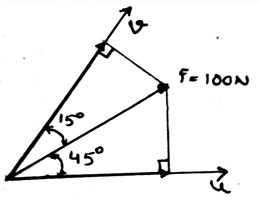Solution:

The component of the force in the u axis, it is equal to 100cos(45˚). We need to consider the triangle and then accordingly apply the trigonometry. This is one of the way of resolving the components.

QUESTION: 2

### For two vectors A and B, what is A.B (if they have angle α between them)?

Solution:

The dot product of the two vectors is always the product of the magnitudes of the two forces and the cosine of the angle between them. We need to consider the triangle and then accordingly apply the trigonometry. This is one of the way of resolving the components.

QUESTION: 3

### Which statement is right?

Solution:

For three vectors A, B and D the various laws are. Communitive law: A.B =B.A. While distributive law is A.(B+D) = (A.B) + (A.D). And multiplication law is a(A.B) = A.(aB).

QUESTION: 4

What is Distributive law?

Solution:

For three vectors A, B and D the various laws are. Communitive law: A.B =B.A. While distributive law is A.(B+D) = (A.B) + (A.D). And multiplication law is a(A.B) = A.(aB).

QUESTION: 5

What is multiplication law?

Solution:

For three vectors A, B and D the various laws are. Communitive law: A.B =B.A. While distributive law is A.(B+D) = (A.B) + (A.D). And multiplication law is a(A.B) = A.(aB).

QUESTION: 6

Which is true for two vector A = A1i + A2j + A3k and B = B1i + B2j + B3k?

Solution:

The multiplication of x, y and z components with their respective same component give a scalar, equal to 1, i.e. i.i = 1 and j.j = 1, while jxj =0. This is the basic principle of the vector algebra which needs to apply wherever needed.

QUESTION: 7

Determine the magnitude of the force F = 300j parallel to the direction of AB?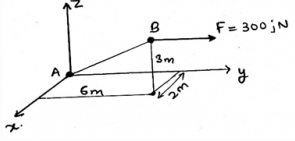Solution:

Force component in the direction parallel to the AB is given by unit vector 0.286i + 0.857j + 0.429k. Now (300j).(0.286i + 0.857j + 0.429k) = 257.1N. Just try to resolve the force into it’s particular components.

QUESTION: 8

What is k.i?

Solution:

As the dot product of only the same Cartesian component is unity, i.e. i.i = 1 and j.j =1, rest all remaining dot product will give 0(i.j = 0 and j.k = 0). Cross product of the same plane vectors always give zero. And dot product of the same plane vector gives a scalar quantity.

QUESTION: 9

What is {(i.i) + (-i.j) + (-k.k) + (k.i)}.(Ai +Bj + Cz)?

Solution:

{(i.i) + (-i.j) + (-k.k) + (k.i)} = 0. As (1 + 0 + 0 – 1). Cross product of the same plane vectors always give zero. And dot product of the same plane vector gives a scalar quantity.

QUESTION: 10

α = cos-1(A.B/AB). What is the range of α?

Solution:

Cosine inverse function is defined only between 0˚ to 180˚. It cannot be defined under any of the given range, because this is the principle range of the inverse cosine function.

QUESTION: 11

What is the value of {(AxB).(C.D)x(AxB).(C.D)}? For the four vectors A, B, C and D, with A, B, C and D all lying in the same plane?

Solution:

Here we are trying to do the cross product of the two vectors in the same plane. Which will give us zero. While dot product of the same plane vector will give a scalar quantity, not zero.

QUESTION: 12

What is (AxB).(BxA); or A = A1i + A2j + A3k and B = B1i + B2j + B3k?

Solution:

Here we are trying to do the cross product of the two vectors in the same plane. Which will give us zero. While dot product of the same plane vector will give a scalar quantity, not zero.

QUESTION: 13

What is the value of θ in the figure given below ?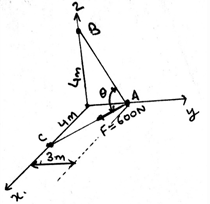Solution:

Fab= -(3/5)j + (4/5)k ; F= (4/5)i – (3/5)j ; θ = cos-1(Fab.F) = 69˚. This is the application of the triangle over the figure. Try to resolve the components of the given force. It will be easy.

QUESTION: 14

Refer to the diagram given below, determine the magnitude of the force F = 300j perpendicular to the direction of AB.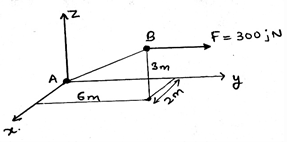Solution:

Force’s component in the direction perpendicular to AB is -73.5i + 80j – 110k N. This is the application of the triangle over the figure. Try to resolve the components of the given force. It will be easy.

QUESTION: 15

Determine the magnitude of the projection of the vector force F = 100N, onto the v axis, from the figure given below.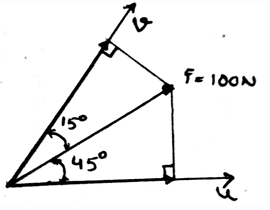Solution:

The component of the force in the v axis, it is equal to 100cos(15˚). This is the application of the triangle over the figure. Try to resolve the components of the given force. It will be easy.

QUESTION: 16

What is the dot product of two vectors which are having magnitude equal to unity and are making an angle of 45°?

Solution:

The dot product of two vectors having the angle between them equal to 45° will have the product of the vector’s magnitude. As the vectors are of unit magnitude, their product will be unity. Thus the magnitude factor would be cosine function at 45°.

QUESTION: 17

Mathematically, for two vectors A and B of any magnitude, the cross product of both, i.e. AxB = given by:

Solution:

The cross product of two vectors gives a vector which is perpendicular to both of the vectors. And the mathematic equation for the same is given by: |A||B|sinØ. And the dot product of the same by any of the other two vectors will give the answer zero, as perpendicular.

QUESTION: 18

Commutative law is valid for the cross product of two vectors. (Commutative law: PxQ = QxP; for two vectors P and Q)

Solution:

This statement is wrong. It is not possible, unless we apply a negative sign to the RHS of the equation. That is PxQ = -(QxP). It is because, if you curl your wrist from one vector towards another vector, the thumb projected will give the direction of the cross product. Thus if you reverse the direction, negative sign is necessary.

QUESTION: 19

Which among the following is the distributive law for the cross product of three vectors?

Solution:

The distributive law works just like the simple multiplication of the constant before the brackets. That is in the equation Px(Q+S) = (PxQ) + (PxS), P is crossed by Q and S both. This is simple as, if we first add the two vectors and then do the cross product or we first do the cross product and the do the sum.

QUESTION: 20

Which statement is true? (For three vectors P, Q and R)

Solution:

The associative law is defined in the cross product of three vectors. This property is though valid for the dot product. But for the cross product, it is not true. It is because, in the dot product the final result is the scalar quantity, but in cross product it is the direction too. Thus the answer.

QUESTION: 21

Which of the following is true?

Solution:

As the mathematic equation for the cross product is having a cosine function in it, in which the angle used in the function is the angle between the vectors. Thus the cross product will be zero if the angle between them is 90.

QUESTION: 22

Which of them is not correct?

Solution:

As asked, the one which is not correct is the third one. The product is containing the cosine function, and the angle which is going to be inserted in the function is the angle between the vectors. Thus if the angle is 90, then the cross will be zero.

QUESTION: 23

The ___________ forces do not cause the rotation.

Solution:

The concurrent forces are the which are somewhere touching the axis of rotation. If any of the force is touching that axis, that force is not considered, or is insufficient to cause a rotation. If a force is concurrent then the perpendicular distance of the force from the line of axis is zero, thus no rotation. As we know rotation is caused by moment.

QUESTION: 24

The tendency of a force to rotate the body is called the moment of the force.

Solution:

The moment of the force about a axis or a point gives the measure of the tendency of the force. It is the cause of the body’s rotation, about that point or at that axis. Thus, the tendency of a force to rotate the body is called the moment of the force.

QUESTION: 25

The moment is the cross product of which two vectors?

Solution:

The cross product needs to take in the proper sequence. If not taken then the answer is just the opposite of the true answer. That’s why, the answer is not the Force and Radius vectors, but the Radius and Force vectors. Because the moment has its direction, as many of the cross products have, and thus precaution needs to be taken.

QUESTION: 26

What is Varigon’s Theorem? (M = Moment, F= Force vector, R= Radius vector)

Solution:

For many vectors acting on the body, Varigon’s Theorem is defined as M = R x F (F = F1 + F2 + F3 + … vectorially adding all the forces). This is just the giving the cross product of individual force and radius vectors and thus the sum is the total moment produced.

QUESTION: 27

What is the mixed triple product of three vectors?

Solution:

It is the scalar expression. It is the dot product of the vector to the vector, which is the result of the cross vector product of two vectors. And if two vectors are held together in the equation by a dot “.”, then the answer which you get is the scalar quantity.

QUESTION: 28

What is the angle made by the vector shown in the figure, with the z-axis?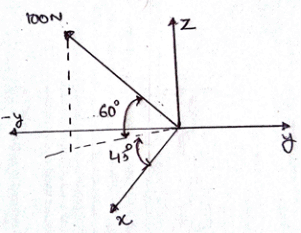Solution:

The question is the tricky one, just the imagination of the viewer is to be enhanced. But is one needs to go with the calculation, one can go and the answer would be 30°. Or simply it is 90° – 60° = 30°.

QUESTION: 29

What is the dot product of the components of the force vector shown in the figure, i.e. the dot product of the z-axis component and the y-axis component of the force?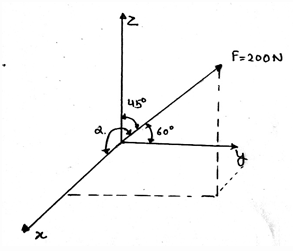Solution:

The dot product of any two vectors is having the cosine function in it. It is being multiplied by product of the magnitudes of the vectors. So if the angle is 90°, then the value of cosine function would be zero, thus 0. And the value of the sine angle is 1, thus the result.

QUESTION: 30

What is the magnitude of the resultant vector’s unit vector? (Resultant vector of the two vectors shown in the figure below)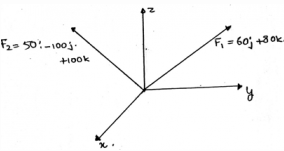Solution:

The question asked is asking for the magnitude of the unit vector of the resultant vector, thus the answer is 1. Whether the magnitude of the vector be any quantity, but the unit vector will have the magnitude equal to unity. And the direction given by the unit vector will be in the same direction as that of the vector.